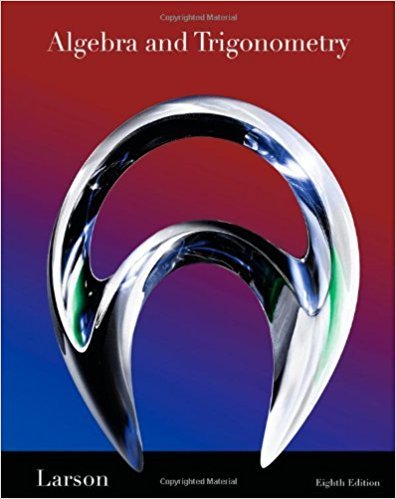×
×

# Solutions for Chapter 6: Trigonometry## Full solutions for Algebra and Trigonometry | 8th Edition

ISBN: 9781439048474Solutions for Chapter 6: Trigonometry

Solutions for Chapter 6
4 5 0 353 Reviews
30
0
##### ISBN: 9781439048474

This expansive textbook survival guide covers the following chapters and their solutions. This textbook survival guide was created for the textbook: Algebra and Trigonometry, edition: 8. Since 141 problems in chapter 6: Trigonometry have been answered, more than 46754 students have viewed full step-by-step solutions from this chapter. Algebra and Trigonometry was written by and is associated to the ISBN: 9781439048474. Chapter 6: Trigonometry includes 141 full step-by-step solutions.

Key Math Terms and definitions covered in this textbook
• Adjacency matrix of a graph.

Square matrix with aij = 1 when there is an edge from node i to node j; otherwise aij = O. A = AT when edges go both ways (undirected). Adjacency matrix of a graph. Square matrix with aij = 1 when there is an edge from node i to node j; otherwise aij = O. A = AT when edges go both ways (undirected).

• Big formula for n by n determinants.

Det(A) is a sum of n! terms. For each term: Multiply one entry from each row and column of A: rows in order 1, ... , nand column order given by a permutation P. Each of the n! P 's has a + or - sign.

• Cholesky factorization

A = CTC = (L.J]))(L.J]))T for positive definite A.

• Elimination.

A sequence of row operations that reduces A to an upper triangular U or to the reduced form R = rref(A). Then A = LU with multipliers eO in L, or P A = L U with row exchanges in P, or E A = R with an invertible E.

• Ellipse (or ellipsoid) x T Ax = 1.

A must be positive definite; the axes of the ellipse are eigenvectors of A, with lengths 1/.JI. (For IIx II = 1 the vectors y = Ax lie on the ellipse IIA-1 yll2 = Y T(AAT)-1 Y = 1 displayed by eigshow; axis lengths ad

• Iterative method.

A sequence of steps intended to approach the desired solution.

• lA-II = l/lAI and IATI = IAI.

The big formula for det(A) has a sum of n! terms, the cofactor formula uses determinants of size n - 1, volume of box = I det( A) I.

• Linear transformation T.

Each vector V in the input space transforms to T (v) in the output space, and linearity requires T(cv + dw) = c T(v) + d T(w). Examples: Matrix multiplication A v, differentiation and integration in function space.

• Nullspace matrix N.

The columns of N are the n - r special solutions to As = O.

• Pivot.

The diagonal entry (first nonzero) at the time when a row is used in elimination.

• Plane (or hyperplane) in Rn.

Vectors x with aT x = O. Plane is perpendicular to a =1= O.

• Projection p = a(aTblaTa) onto the line through a.

P = aaT laTa has rank l.

• Rank one matrix A = uvT f=. O.

Column and row spaces = lines cu and cv.

• Rank r (A)

= number of pivots = dimension of column space = dimension of row space.

• Rotation matrix

R = [~ CS ] rotates the plane by () and R- 1 = RT rotates back by -(). Eigenvalues are eiO and e-iO , eigenvectors are (1, ±i). c, s = cos (), sin ().

• Saddle point of I(x}, ... ,xn ).

A point where the first derivatives of I are zero and the second derivative matrix (a2 II aXi ax j = Hessian matrix) is indefinite.

• Similar matrices A and B.

Every B = M-I AM has the same eigenvalues as A.

• Singular Value Decomposition

(SVD) A = U:E VT = (orthogonal) ( diag)( orthogonal) First r columns of U and V are orthonormal bases of C (A) and C (AT), AVi = O'iUi with singular value O'i > O. Last columns are orthonormal bases of nullspaces.

• Subspace S of V.

Any vector space inside V, including V and Z = {zero vector only}.

• Symmetric matrix A.

The transpose is AT = A, and aU = a ji. A-I is also symmetric.

×